Home > PC > Chapter 11 > Lesson 11.1.4 > Problem11-55

11-55.
1. Simplify the following expressions. Write your answers in a + bi form. Homework Help ✎

1. (4 + 3i) − (2 − 4i)

2. (4 − 2i) (3 + 4i)

3. (3 − 5i)2

4.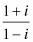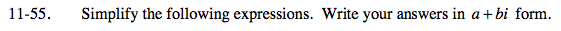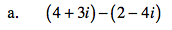4 + 3i − 2 + 4i

Now combine like terms.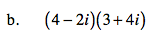Use the Ditributive Property. You should have four terms before combining like terms and simplifying.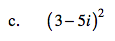(3 − 5i)(3 − 5i)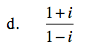$\frac{1+i}{1-i}\left( \frac{1+i}{1+i}\right)$